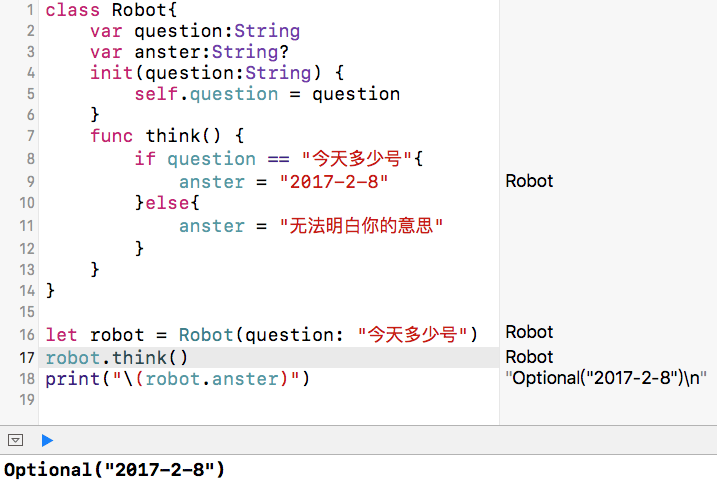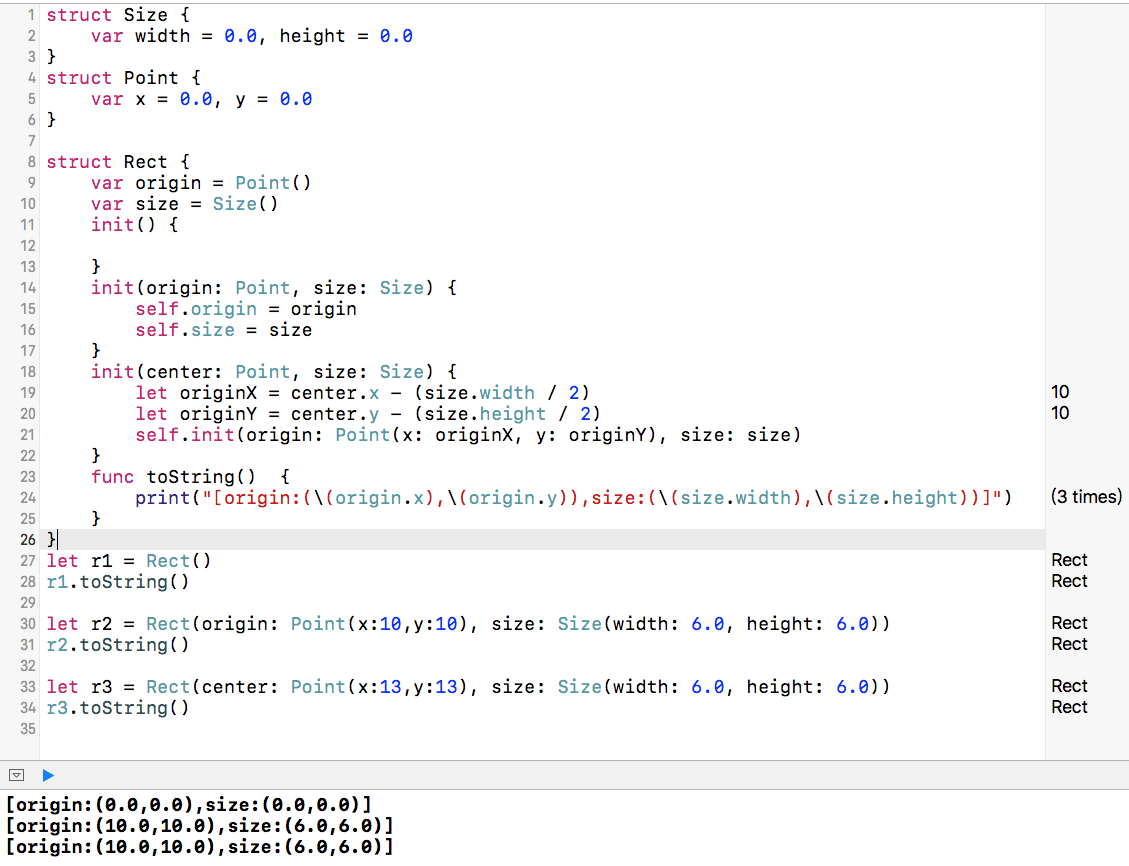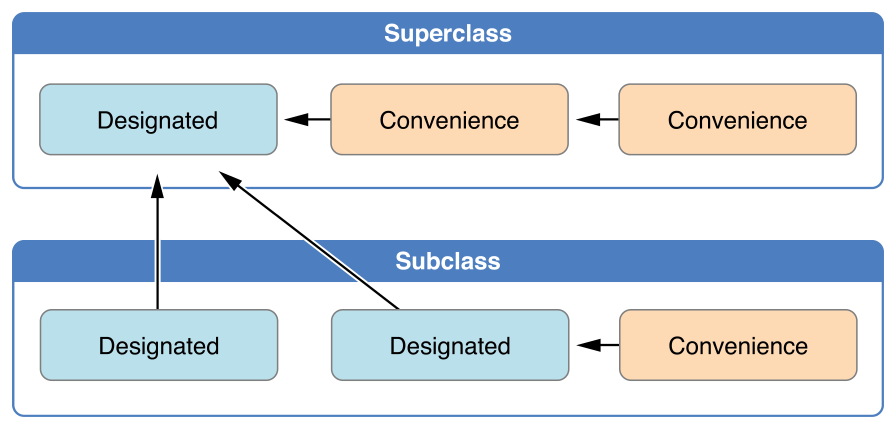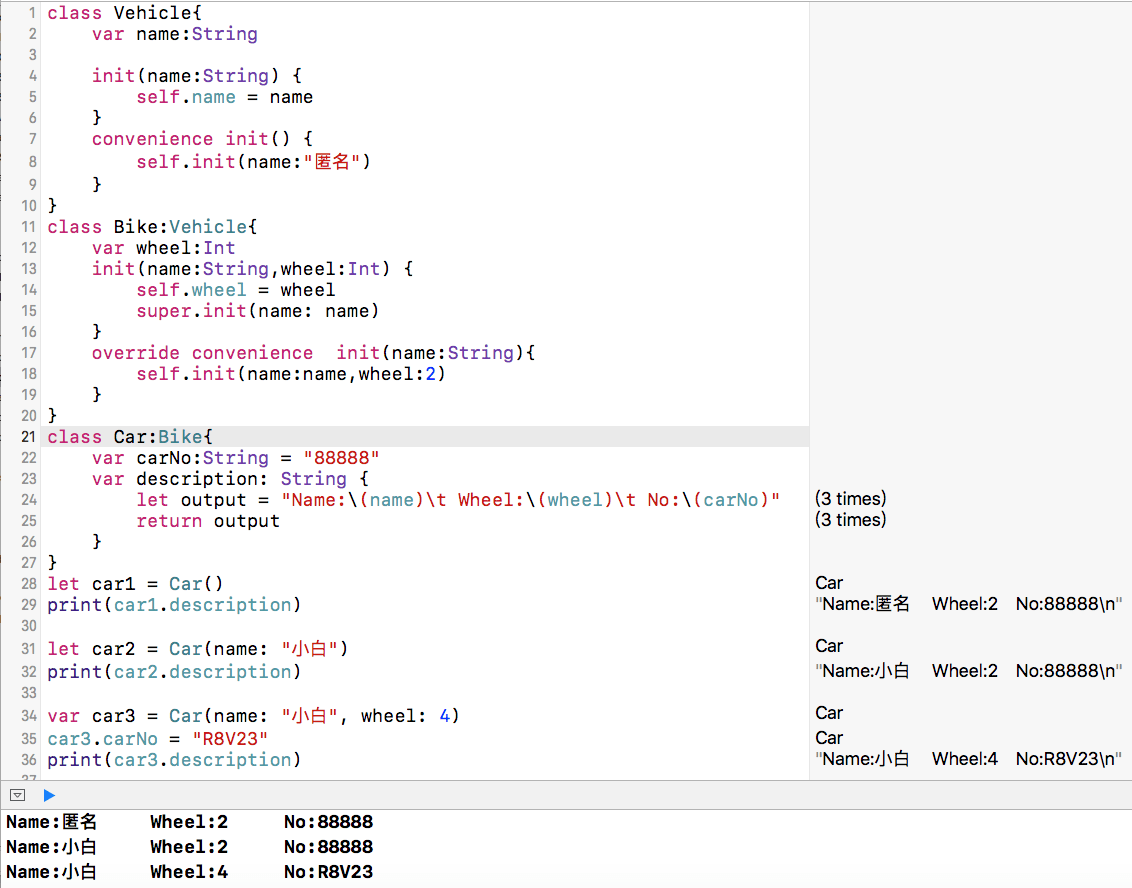# 设置存储属性的初始值

``````struct Point{
var x:Int,y:Int
init() {
x = 0
y = 0
}
}
``````

``````struct Point{
var x:Int=0,y:Int=0
}
``````

# 自定义初始化

``````class Color{
var red:Int,green:Int,blue=0
init(_ red:Int) {
self.red = red
self.green = 0
}
init(red:Int,withGreen green:Int) {
self.red = red
self.green = green
}
init(red:Int,green:Int,blue:Int) {
self.red = red
self.green = green
self.blue = blue
}
func toString() {
print("(r:\(self.red),g:\(self.green),b:\(self.blue))")
}
}

let color1 = Color(10)
color1.toString() //打印结果：(r:10,g:0,b:0)
let color2 = Color(red:10,withGreen:20)
color2.toString() //打印结果：(r:10,g:20,b:0)
let color3 = Color(red: 10, green: 20, blue: 30)
color3.toString() //打印结果：(r:10,g:20,b:30)
``````

``````class Robot{
var question:String
var anster:String?
init(question:String) {
self.question = question
}
func think() {
if question == "今天多少号"{
anster = "2017-2-8"
}else{
anster = "无法明白你的意思"
}
}
}

let robot = Robot(question: "今天多少号")
robot.think()
print("\(robot.anster)")
``````# 默认构造器

``````class Color{
var red:Int=255,green:Int=255,blue=0
}
let color = Color()
``````

``````struct Point{
var x:Int,y:Int
}
let point = Point(x: 10, y: 20)
``````

# 值类型的构造器代理

``````struct Size {
var width = 0.0, height = 0.0
}
struct Point {
var x = 0.0, y = 0.0
}

struct Rect {
var origin = Point()
var size = Size()
init() {

}
init(origin: Point, size: Size) {
self.origin = origin
self.size = size
}
init(center: Point, size: Size) {
let originX = center.x - (size.width / 2)
let originY = center.y - (size.height / 2)
self.init(origin: Point(x: originX, y: originY), size: size)
}
func toString()  {
print("[origin:(\(origin.x),\(origin.y)),size:(\(size.width),\(size.height))]")
}
}
let r1 = Rect()
r1.toString()

let r2 = Rect(origin: Point(x:10,y:10), size: Size(width: 6.0, height: 6.0))
r2.toString()

let r3 = Rect(center: Point(x:13,y:13), size: Size(width: 6.0, height: 6.0))
r3.toString()
``````# 类的继承和初始化

• 指定构造器必须调用直接超类（如果有超类）的指定构造器。
• 便捷构造器只能调用同一个类中的其他构造器。
• 便捷构造器必须以调用指定构造器作为结束。• 如果子类没有定义指定构造器，自动继承超类的所有指定构造器。
• 如果子类提供了超类所有指定构造器的实现，则它会自动继承超类的所有便捷构造器。

``````class Vehicle{
var name:String

init(name:String) {
self.name = name
}
convenience init() {
self.init(name:"匿名")
}
}
class Bike:Vehicle{
var wheel:Int
init(name:String,wheel:Int) {
self.wheel = wheel
super.init(name: name)
}
override convenience  init(name:String){
self.init(name:name,wheel:2)
}
}
class Car:Bike{
var carNo:String = "88888"
var description: String {
let output = "Name:\(name)\t Wheel:\(wheel)\t No:\(carNo)"
return output
}
}
let car1 = Car()
print(car1.description)

let car2 = Car(name: "小白")
print(car2.description)

var car3 = Car(name: "小白", wheel: 4)
car3.carNo = "R8V23"
print(car3.description)
``````# 示例代码

https://github.com/99ios/23.16